Ex 2.1

Chapter 2 Class 12 Inverse Trigonometric Functions
Serial order wise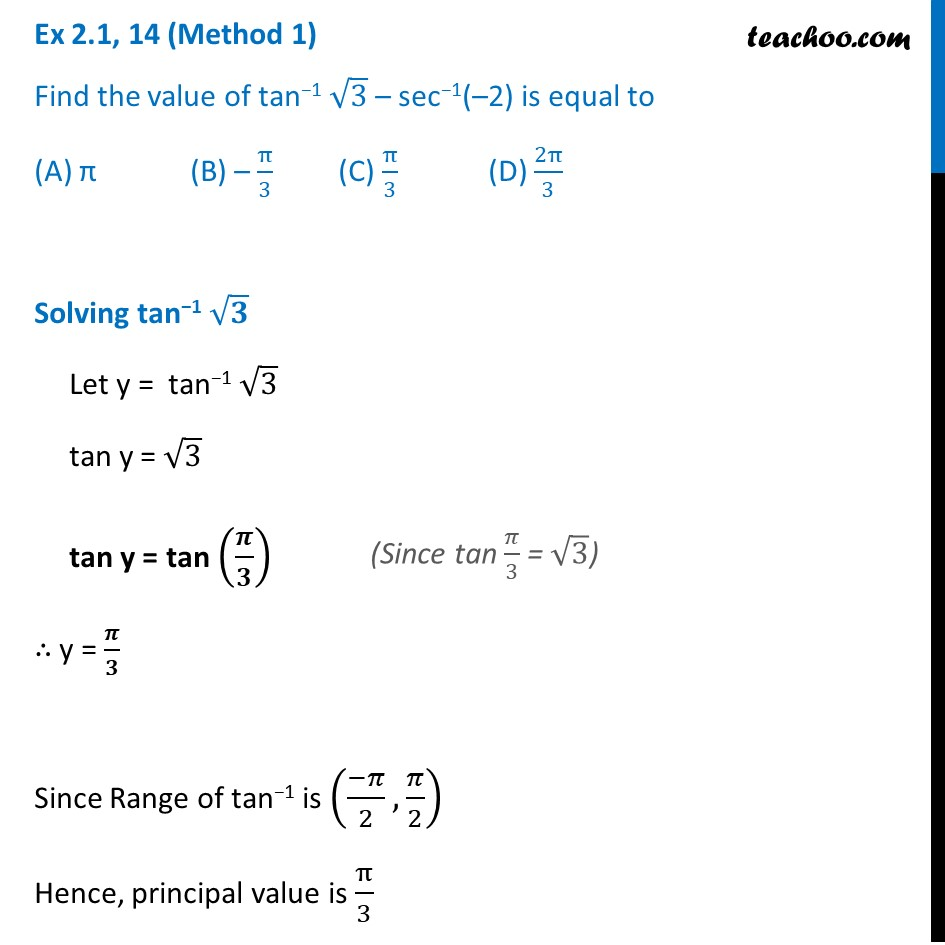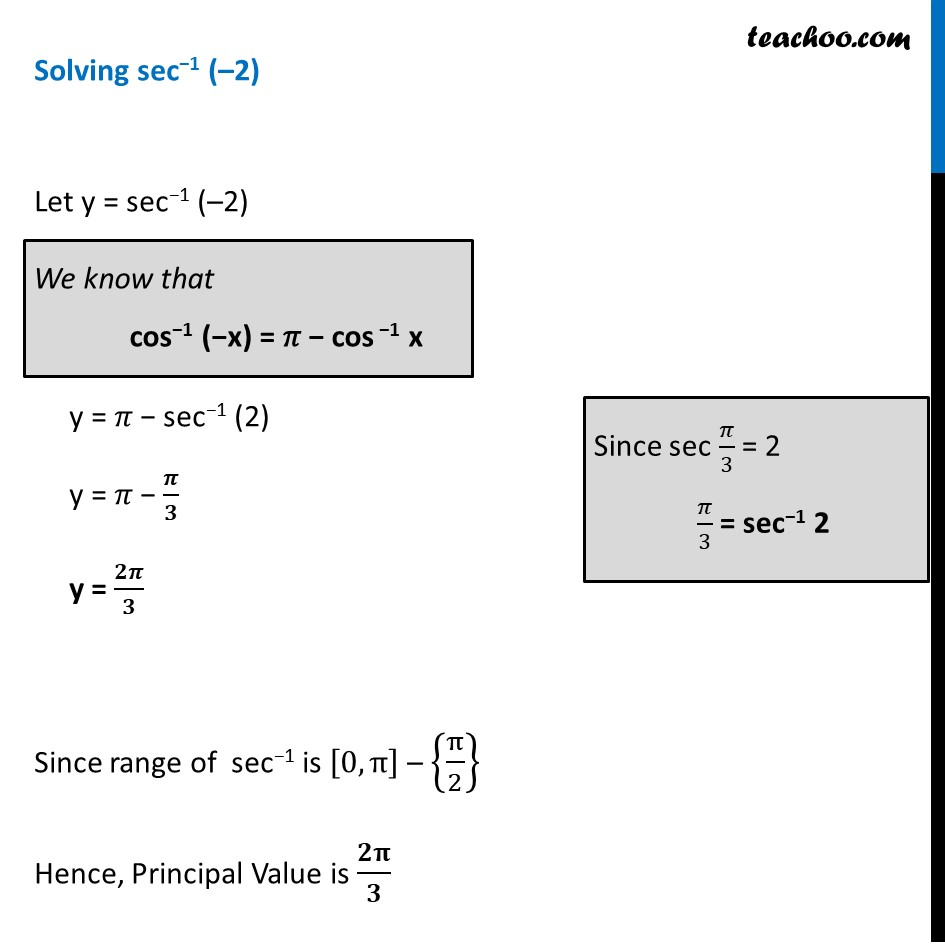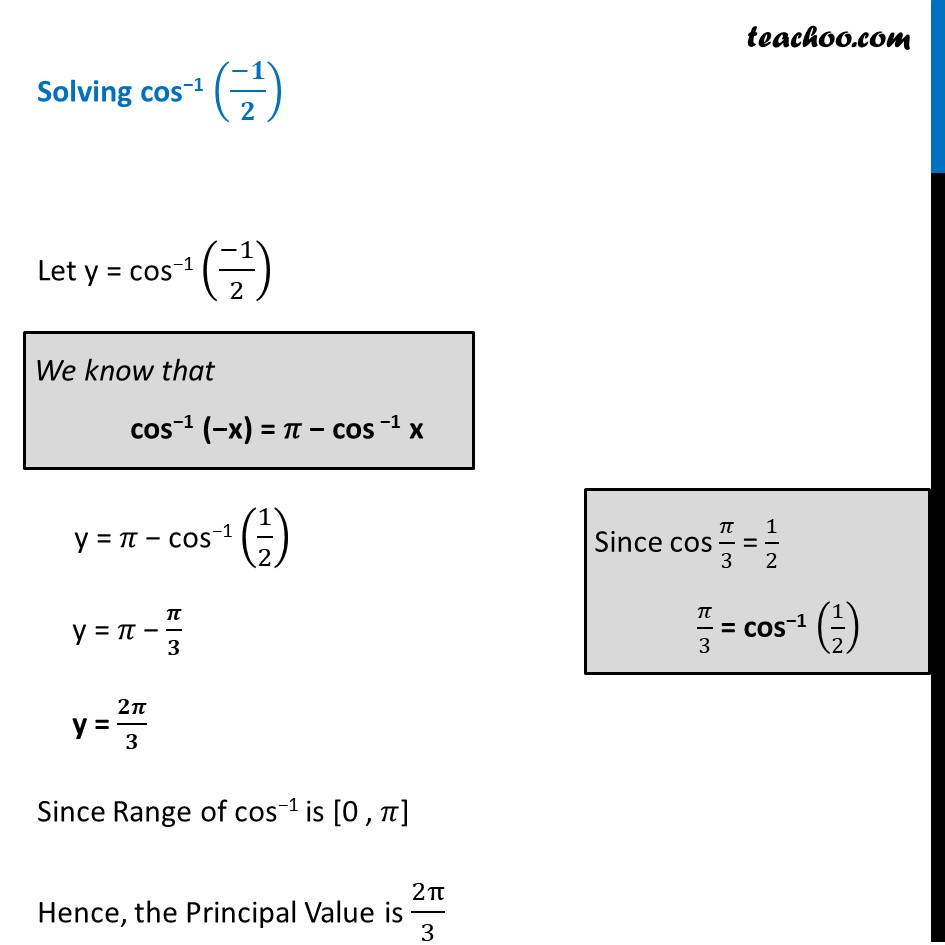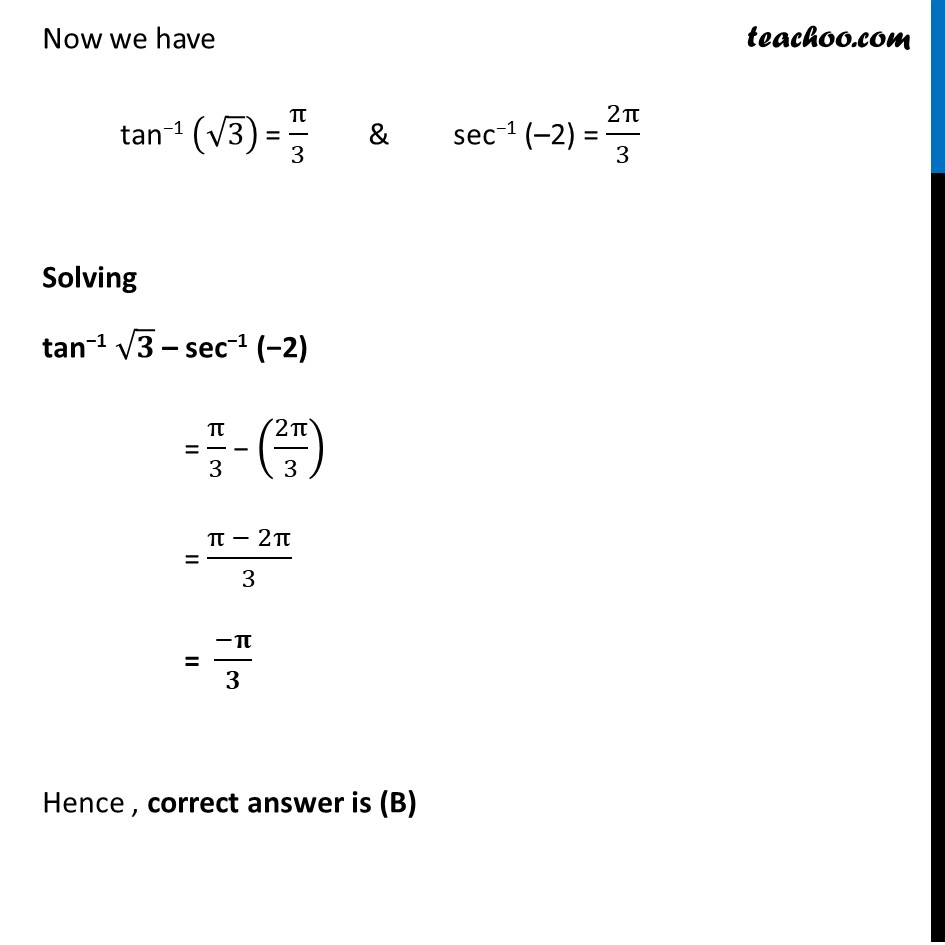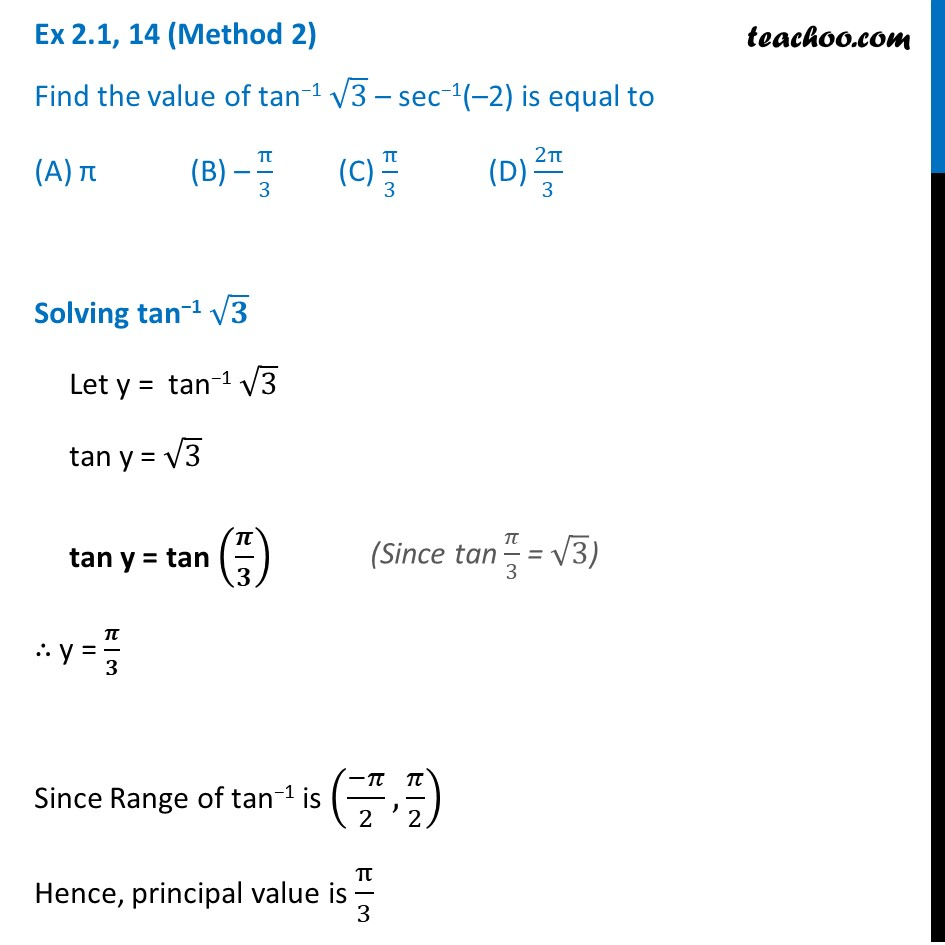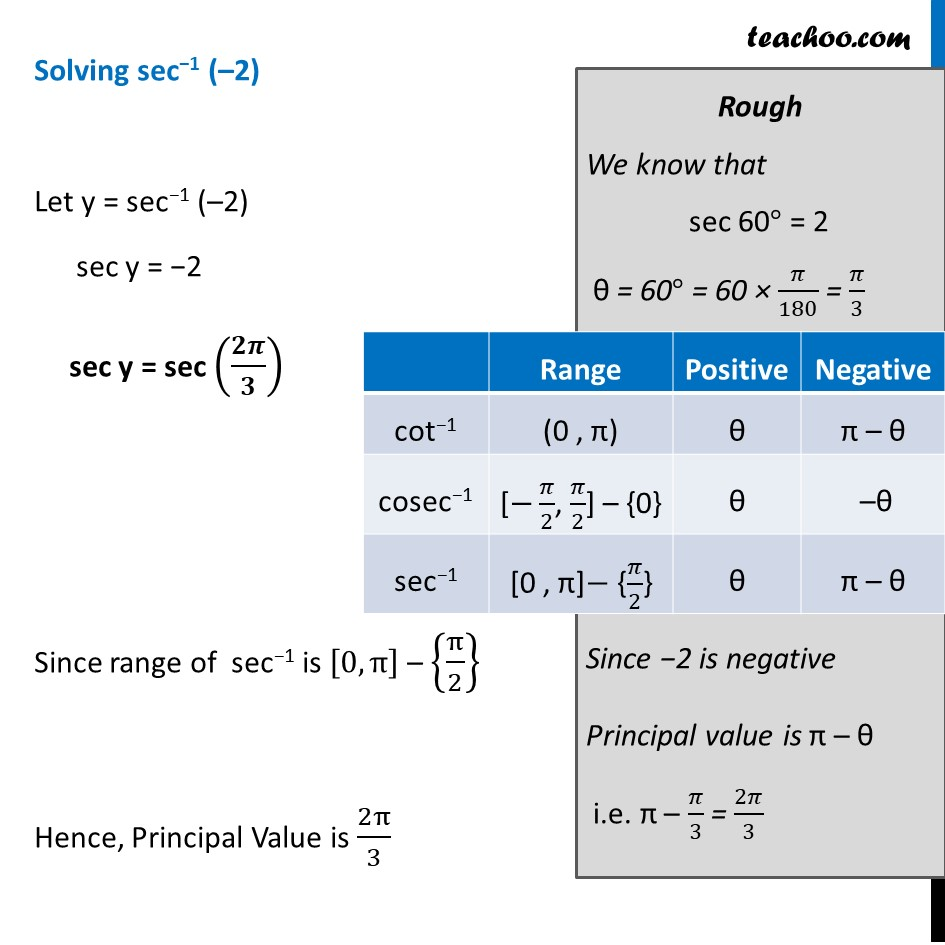Learn in your speed, with individual attention - Teachoo Maths 1-on-1 Class

### Transcript

Ex 2.1, 14 (Method 1) Find the value of tan−1 √3 – sec−1(–2) is equal to π (B) – π/3 (C) π/3 (D) 2π/3 Solving tan−1 √𝟑 Let y = tan−1 √3 tan y = √3 tan y = tan (𝝅/𝟑) ∴ y = 𝝅/𝟑 Since Range of tan−1 is ((−𝜋)/2,𝜋/2) Hence, principal value is π/3 Solving sec−1 (–2) Let y = sec−1 (–2) y = 𝜋 − sec−1 (2) y = 𝜋 − 𝝅/𝟑 y = 𝟐𝝅/𝟑 Since range of sec−1 is [0,π] – {π/2} Hence, Principal Value is 𝟐𝛑/𝟑 We know that cos−1 (−x) = 𝜋 − cos −1 x Since sec a𝜋/3 = 2 𝜋/3 = sec−1 2 Solving sec−1 (–2) Let y = sec−1 (–2) y = 𝜋 − sec−1 (2) y = 𝜋 − 𝝅/𝟑 y = 𝟐𝝅/𝟑 Since range of sec−1 is [0,π] – {π/2} Hence, Principal Value is 𝟐𝛑/𝟑 We know that cos−1 (−x) = 𝜋 − cos −1 x Since sec a𝜋/3 = 2 𝜋/3 = sec−1 2 Solving cos−1 ((−𝟏)/𝟐) Let y = cos−1 ((−1)/2) y = 𝜋 − cos−1 (1/2) y = 𝜋 − 𝝅/𝟑 y = 𝟐𝝅/𝟑 Since Range of cos−1 is [0 , 𝜋] Hence, the Principal Value is 2π/3 We know that cos−1 (−x) = 𝜋 − cos −1 x Since cos 𝜋/3 = 1/2 𝜋/3 = cos−1 (1/2) Now we have tan−1 (√3) = π/3 & sec−1 (–2) = 2π/3 Solving tan−1 √𝟑 – sec−1 (−2) = π/3 − (2π/3) = (π − 2π)/3 = (−𝛑)/𝟑 Hence , correct answer is (B) Ex 2.1, 14 (Method 2) Find the value of tan−1 √3 – sec−1(–2) is equal to π (B) – π/3 (C) π/3 (D) 2π/3 Solving tan−1 √𝟑 Let y = tan−1 √3 tan y = √3 tan y = tan (𝝅/𝟑) ∴ y = 𝝅/𝟑 Since Range of tan−1 is ((−𝜋)/2,𝜋/2) Hence, principal value is π/3 Solving sec−1 (–2) Let y = sec−1 (–2) sec y = −2 sec y = sec (𝟐𝝅/𝟑) Since range of sec−1 is [0,π] – {π/2} Hence, Principal Value is 2π/3 Now we have tan−1 (√3) = π/3 & sec−1 (–2) = 2π/3 Solving tan−1 √𝟑 – sec−1 (−2) = π/3 − (2π/3) = (π − 2π)/3 = (−𝛑)/𝟑 Hence , correct answer is (B)